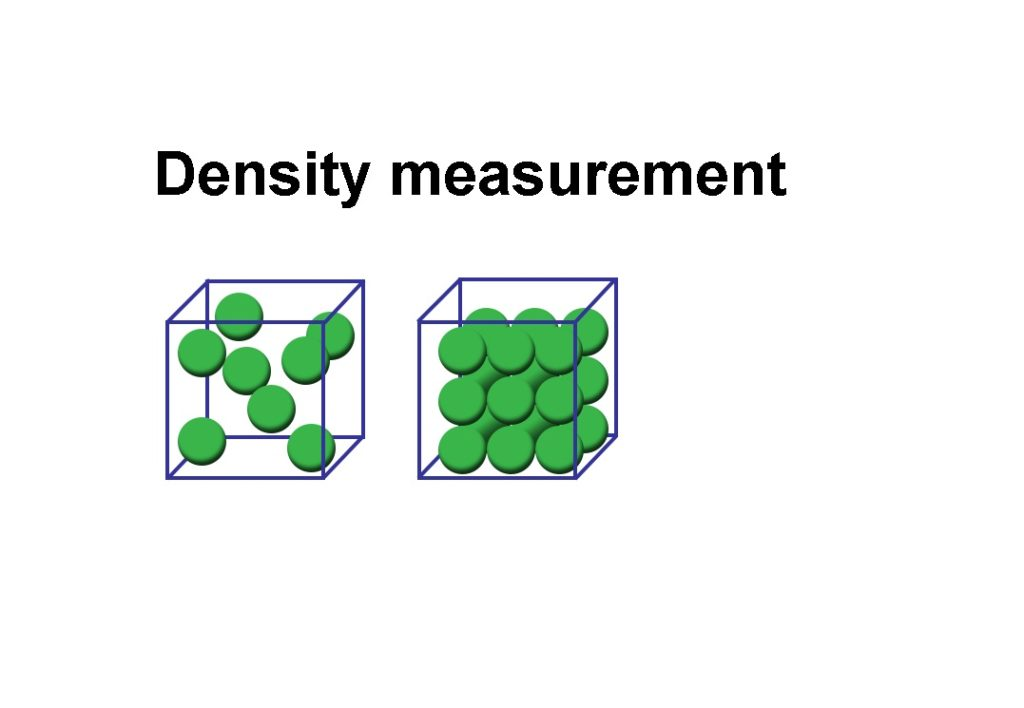# Different methods of Density Measurement## Introduction

Density is defined as the mass per unit volume. Specific gravity is a unitless measurement. It is the ratio of the density of a substance to the density of water, at a standard temperature. This term is also referred to as the relative density.
The measurement and control of liquid density can be quite critical in industrial processes. Density measurement gives useful information about composition, concentration of chemicals or of solids in suspension.

Density can be measured in a number of similar ways to level:

– Hydrostatic pressure
– Vibration
– Differential pressure

The Coriolis mass flowmeter is also capable of performing density measurement.

### Hydrostatic Pressure

This type of density measurement relies on a constant height of liquid and measures the pressure differences. Since level may vary, the principle of operation works on the difference in pressure between any two fixed elevations below the surface. Because the height between these two points does not change, any change in pressure is due to density variations. The distance between these points is equal to the difference in liquid head pressure between these elevations.Density measurement by radiation is based on the increased absorption of gamma radiation for an increase in specific gravity of the material being measured. The main components of such a system are a constant gamma source (usually radium) and a detector. Variations in radiation passing through a fixed volume of flowing liquid is converted into a proportional electrical signal by the detector.This type of measurement is often used in dredging where the density of the mud indicates the effectiveness of the dredging vessel

### Vibration

Damping of a vibrating object in a fluid will increase as the density of the fluid increases. An object is vibrated from an external energy source. The object may be an immersed reed or plate.
Density is measured from one of two procedures:
1. Changes in the natural frequency of vibration can be measured when the object is energised constantly.
2. Changes in the amplitude of vibration can be measured when the object is struck periodically, like a bell

### Differential Pressure

Constant level overflow tanks are the simplest for measuring as only one differential pressure transmitter is required. However applications with level or static pressure variations require compensation.### Temperature Effects

Increases in temperature cause expansion of a liquid, altering its density. Not all liquids expand at the same rate. A specific gravity measurement must be corrected for temperature effects in order to be completely accurate in terms of reference conditions for density and concentration, although in most cases this is not practical. In applications where specific gravity is extremely critical, it is possible to control the temperature to a constant value. The necessary correction to the base temperature can then be included in the density instrument calibration